# SAT II Chemistry : Stoichiometry with Reactions

## Example Questions

### Example Question #9 : Stoichiometry

Octane,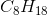, is an important of component of gasoline, which can be burned as fuel in cars. The following is an unbalanced equation for the combustion of octane.

If 114 g of octane are burned in the complete combustion reaction shown below, how many grams of water will be produced?

___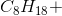___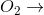___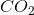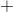___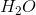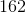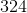Explanation:

First, the equation should be balanced. This can be done with the coefficients 2, 25, 16, and 18.

Next, calculating the molar mass of octane shows that it has a molar mass of 114 g/mol. This means that 1 mole of octane is used in the reaction, and 9 times as many moles of water should be produced, meaning one of the products will be 9 mol of water. Water has a molar mass of 18 g/mol, so the total mass of water produced is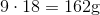.

### Example Question #1 : Stoichiometry With Reactions

Suppose that 4 moles of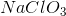undergoes complete decomposition. At STP, how many liters of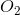would be produced as product? (At STP, one mole of a gas takes up 22.4 L of space.)Explanation:

Start by creating a skeleton equation for the decomposition reaction: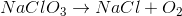This can be balanced to give: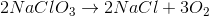This means that 4 mol of reactant would produce 6 mol of oxygen gas. The question states that at STP, one mole of a gas takes up 22.4 L of space, so it is easy to find that 6 moles of oxygen gas would occupy a volume of 134.4 L: